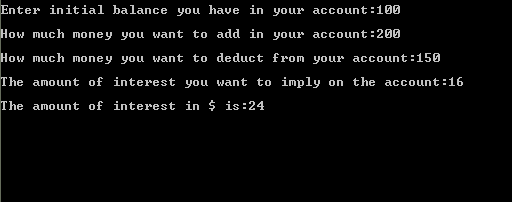# Applying inheritance in C++ programs

In this tutorial you will learn: 1. How to use inheritanace in program? 2. The advantages of inheritance? 3. How base and derived class functions work together? 4. C++ syntax As told before inheritance is very useful in terms of reliability and reuse of the code. In this part of the tutorial I have made a small program to demonstrate how can we make better use of the inheritance by implementing it in some practical situation like account management of some client of a bank. There are two type of accounts in bank, one is the current account and other one is the savings account. The current account only provides the features of credit(adding money) and debit( deducting money) from the account while the saving account also provide users with a feature of interest rate, in this case some amount of money according to the interest percentage is given to the customer above his actual amount(balance). Saving account class is also the current account as we can debit and credit the money from it as well so we can make the current account as the base class while the savings account class as the derived class(because additional functionality is added in the saving account). Here current account is named as “Account” while saving account as “SavingsAccount”. Lets start with the code. strong>Inheritance Basic Step: Open Dev C++ then File > new > source file and start writing the code below.
`#include<iostream>#include<conio.h>using namespace std;`
These are the basic header files which are required for C++ programming. Using namespace std is used at the top of the program because if its not written then we have to write ‘std::’ with every line of the program. #include includes the C++ built in libraries and iostream is used for input and output of the program. Conio.h is used to show output on the screen, if it is not present , the screen flashes and disappears hence not showing output.
`class Account{  private:	double balance; public:	 Account()	 {		 balance=0;	 }	 Account(int x)	 {         balance=x;	 }	 void setBalance()	 {		 cout<<"\nEnter initial balance you have in your account:";		 int x;		 cin>>x;	 balance=x;	 }	 void credit()     {   		 cout<<"\nHow much money you want to add in your account:";		 int x;		 cin>>x;		 balance=balance+x;	 }	 void debit()	 {   cout<<"\nHow much money you want to deduct from your account:";		 int x;		 cin>>x;		 balance=balance-x;                }	 double getBalance()	 {		 return balance;	 } };`
The first class in the program we have is the base class named “Account”, as it is the current account so it contains only one data member that is “balance”. The functions of this class are the constructor, debit, credit and getBalance. Debit function adds some amount(provided by the user) to the original balance while the debit function deducts some amount which user want to remove from his account. Both function have the return type void because they are just adding and subtracting value not returning anything, Another function is the getBalance function which returns the amount of balance currently present in the account of the person. Its return type is double(which is that of balance).
`class SavingsAccount:public Account{   private:	double interestRate; public: 	 SavingsAccount()	 {   		 Account::setBalance();		 interestRate=0;	 }	 void setInterest()	 { cout<<"\nThe amount of interest you want to imply on the account:";	   int y;	   cin>>y;	   interestRate=y;	 }	 double calculateInterest()         {                   cout<<"\nThe amount of interest in \$ is:";		 return((interestRate/100)*getBalance()); 	 }};`
In this code we are working on our derived class “SavingsAccount” which is publicly derived from the base class “Accounts”, it has a data member interest rate. The interest is the only additional feature present in the savingsAccount and it also makes savingAccounts different from the current account as also explained before. So in this class we have a constructor(default) which set the interest rate equal to zero and the base class constructor is called using binary scope resolution operator which sets the balance as input by the user. Next we have functions setInterest and calculateInterest in which user inputs interest she would get from the bank and the calculated interest after multiplication is returned respectively. In calaculate interest function we have divided the interest rate by hundred because the user inputs the interest rate in the form of percentage so this conversion is necessary. getBalance function of the base class is used in the calculate interest function because as already told before that the private data members of the base class can only be accessed by the functions of derived class using the public functions of base class.
`void main(){SavingsAccount s;s.credit();s.debit();s.setInterest();cout<<s.calculateInterest();getch();}`
In the main of the program we have just made an object of the derived class so that all the aspects of the base class are also covered. Then we called different functions of the base class on the derived class object to demonstrate that derived class object can access function of base class object. Finally we displayed the result after finding the interest on the balance as shown in the output. Basic Step: Open Dev C++ then File > new > source file and start writing the code below. Execute > compile then Execute > run Output